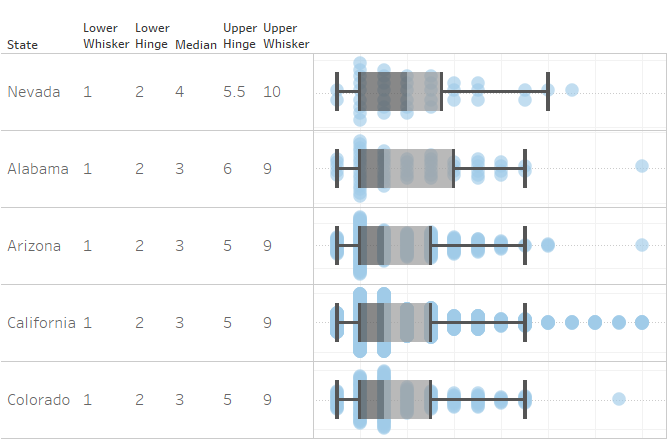## How to do Box Plot Calculations in Tableau

Box plots are great for displaying distribution and in Tableau they’re incredibly easy to make. In fact the simplest box plot in Tableau takes only 4 clicks. Click a dimension, hold ctrl & click a measure, click the “Show Me” tab and select the box plot function and there you go, you have a box plot!### The Constituent Parts

Box plots are made of five key components: the median, the upper and lower hinges, and the upper and lower whiskers. The median is a standard statistical measure and is included in Tableau’s library of formulae, it’s the 50th percentile value, the middle number in a data set. The hinges however are harder to work out because they are near the 25th and 75th percentiles but not there exactly and the distance away from these values depends on the size of the data set.

### Tukey Hinges

The hinges Tableau uses are Tukey inclusionary hinges, so named after John Tukey the person who first created box plots. Tukey hinges are the midway points in the first and second halves of data. If there are an even number of data points then the data is split straight down the middle. However if there are an odd number of data points then the median value is duplicated and used by both halves. This is illustrated below where the number sets of 1-5, 1-7, and 1-9 each duplicate their median value. Hinges are then the medians of their respective halves of the data.

### The Whiskers

The whiskers then stretch out to the furthest data points within 1.5 interquartile ranges of the hinges, where the interquartile range [IQR] is the distance between the two hinges.

### So How Do We Calculate This?

First of all, the data being plotted needs to be at the most granular level. This method uses table calculations that requires index formulae computed using the measure itself so before you start, untick the “Aggregate Measures” in the analysis menu. Now for the calculations coming, we are using Superstore data to generate box plots of Quantity by State to create the view below. When you want tailor them to your own calculations, just replace any references to Quantity with your measure of choice and make sure each of your table calculations are computed using it. If you can’t see Quantity as an option for ‘compute by’, make sure the measure is not aggregated and set it as a continuous dimension.

### Lower Hinge

```WINDOW_MEDIAN( SUM([Quantity]), FIRST(), INT((WINDOW_COUNT(SUM([Quantity]))+1)/2) - INDEX() )```

The first part of the window median says that we are taking the median of quantity values, the middle part says the range is starting with the first value, and the last part anchors the end of the range in the middle of the data set, inclusive of the median.

### Upper Hinge

```WINDOW_MEDIAN( SUM([Quantity]), INT(ROUND((WINDOW_COUNT(SUM([Quantity]))+1)/2,0)) - INDEX(), LAST() )```

The first part of the window median says that we are taking the median of quantity values, the middle part says the range is starting in the middle of the data set, inclusive of the median, and the last part anchors the end of the range in the middle of the data set, inclusive of the median.

### IQR (Interquartile Range)

This calculation is a good descriptor of the spread of a data set and also helps to make the whisker calculations clearer.

`[Upper Hinge] - [Lower Hinge]`

### Lower Whisker

`WINDOW_MIN( `
`IF SUM([Quantity]) > [Lower Hinge] - 1.5*[IQR] OR SUM([Quantity]) = [Lower Hinge]`
`THEN SUM([Quantity])`
`END`
`)`

This takes the minimum value for any data within 1.5 IQRs of the lower hinge or the lower hinge itself.

### Upper Whisker

`WINDOW_MAX(`
`IF SUM([Quantity]) < [Upper Hinge] + 1.5*[IQR] OR SUM([Quantity]) = [Upper Hinge]`
`THEN SUM([Quantity])`
`END`
`)`

This takes the maximum value for any data within 1.5 IQRs of the upper hinge or the upper hinge itself.

### Sorting

Now for a final touch of flair, if you want to sort your dimension by these values create an integer parameter from 1 to 5 for the statistics and another from 1 to 2 for ascending or descending and then create a sorting calculation. This new calculation then needs to go before your first dimension with its header hidden. The calculation should be roughly as below, also computed along your designated measure.

```CASE [Ascending or Descending] WHEN 1 THEN CASE [Sort States by] WHEN 1 THEN [Lower Whisker] WHEN 2 THEN [Lower Hinge] WHEN 3 THEN [Median] WHEN 4 THEN [Upper Hinge] WHEN 5 THEN [Upper Whisker] END WHEN 2 THEN CASE [Sort States by] WHEN 1 THEN -[Lower Whisker] WHEN 2 THEN -[Lower Hinge] WHEN 3 THEN -[Median] WHEN 4 THEN -[Upper Hinge] WHEN 5 THEN -[Upper Whisker] END END```

And there you go, that’s box plot calculations in Tableau!

London, UK

### 3 thoughts on “How to do Box Plot Calculations in Tableau”

1.Uday says:

This Very Valuable! Thanks, Ian.

2.Laura says:

It took me a while to get the “Compute By” order just right as I have a few more dimensions in my view than you did, but it worked and I’d have never figured it out without this!!! You’re my Tableau hero!!! 🙂

3.Mark says:

I’m new to Tableau coming from Spotfire and even though the above described does the trick of displaying some additional statistical information for each box (thanks!) I feel this is too complicated for the typical analyst. In Spotfire all of this is readily available by a mouse click. Is there no such thing in Tableau and you really have to do this manually?